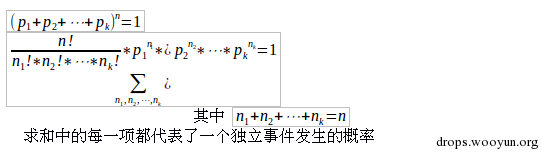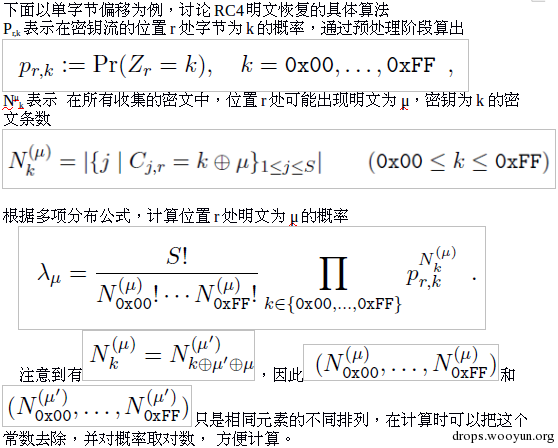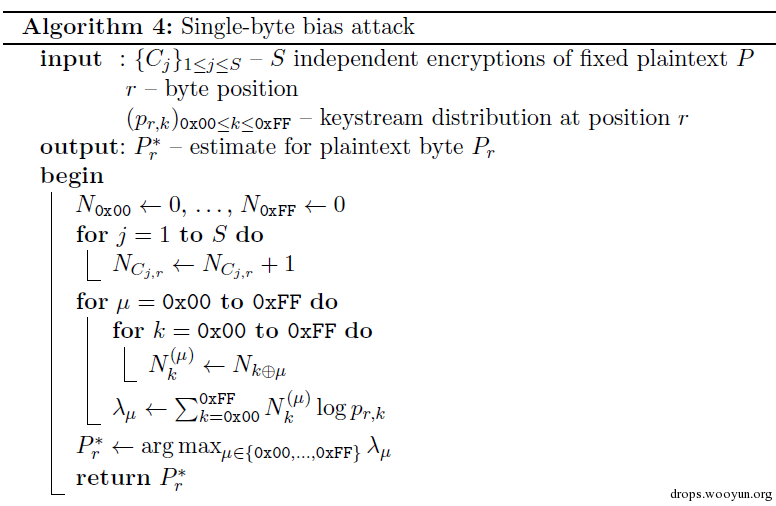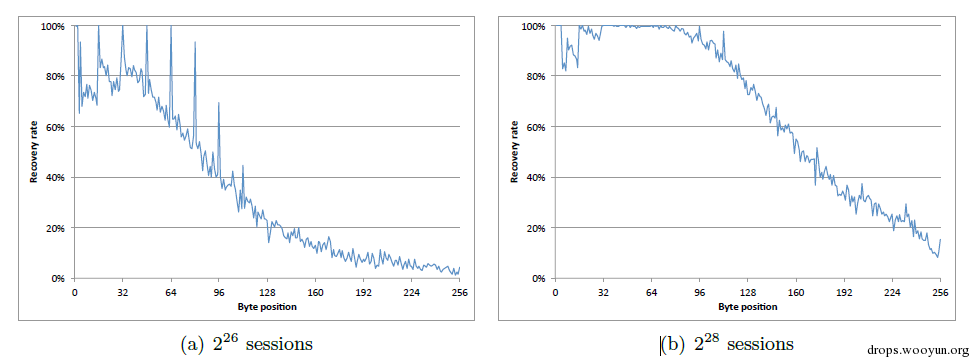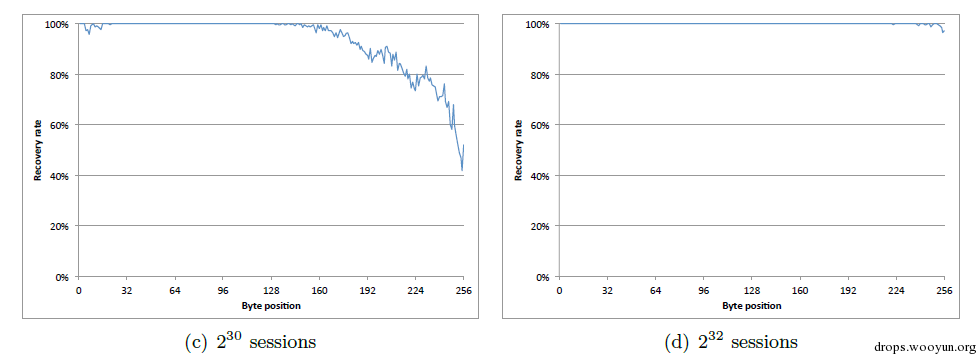# 0x00背景

RC4是美国密码学家Ron Rivest在1987年设计的密钥长度可变的流加密算法。它加解密使用相同的密钥，因此也属于对称加密算法。RC4曾被用在有线等效加密（WEP）中，但由于其错误的使用的方式已被有效破解，而如今，它又被TLS协议所放弃。

# 0x01 RC4

RC4算法分为两个部分。第一部分是密钥调度算法（key-scheduling algorithm）,根据key初始化S盒，第二部分是伪随机数生成算法(Pseudo-random generation algorithm)，生成密钥流，同时更新S盒的状态。

``````Input： 密钥K ，K长度Ｌ字节
Output:初始化后的S盒子
For i=0 to 255 do
S[i] = i
j=0
for i=0 to 255 do
j = ( j+S[i]+key[i mod L] )mod 256
Swap(S[i],S[j])
Return S
``````

``````Input: S盒
Output：生成的密钥流
i=0
j=0
while GeneratingOuput
I = (i+1) mod 256
j = (j+S[i]) mod 256
Swap（S[i],S[j]）
Z = S[ (S[i]+S[j]) mod 256]
Output z
``````

# 0x02 偏移

Rc4的最初若干字节密钥流的非均匀分布

## 1. 单字节偏移

RC4单字节偏移现象最初由Mantin and Shamir等人发现。他们指出密钥流的第二个字节,Z2=0的概率约为1/128,而不是1/256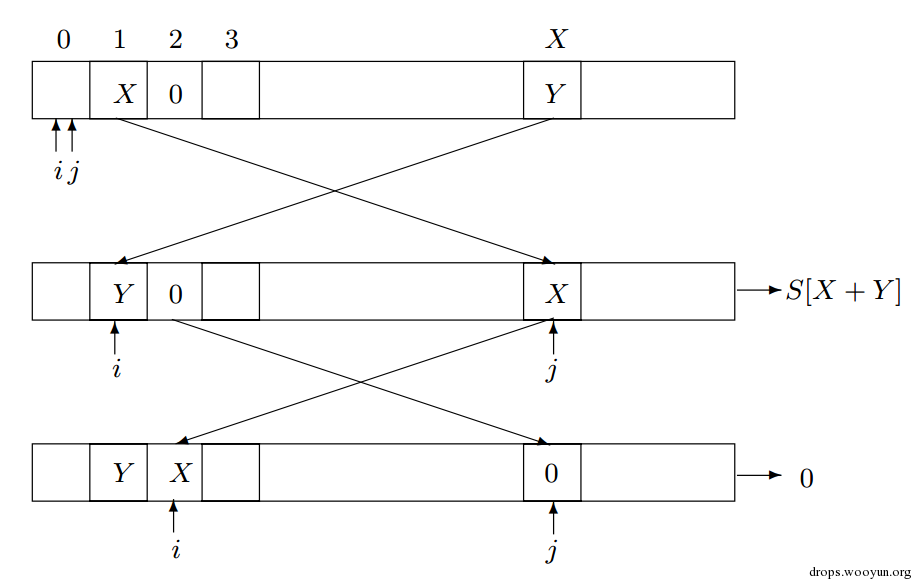``````P[z2 = 0] =  P[z2 = 0|S= 0] * P[S= 0]
+ P[z2 = 0|S ≠ 0] * P[S ≠ 0]
≈ 1*1/256 + 1/256*(1-1/256)
≈ 1/128
``````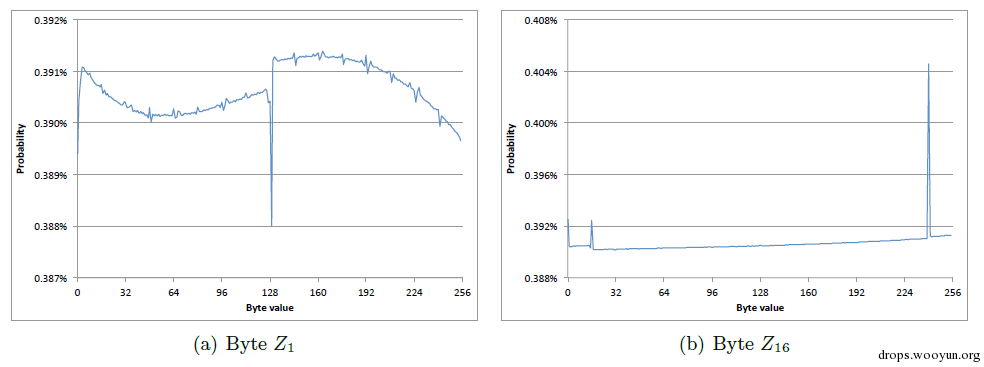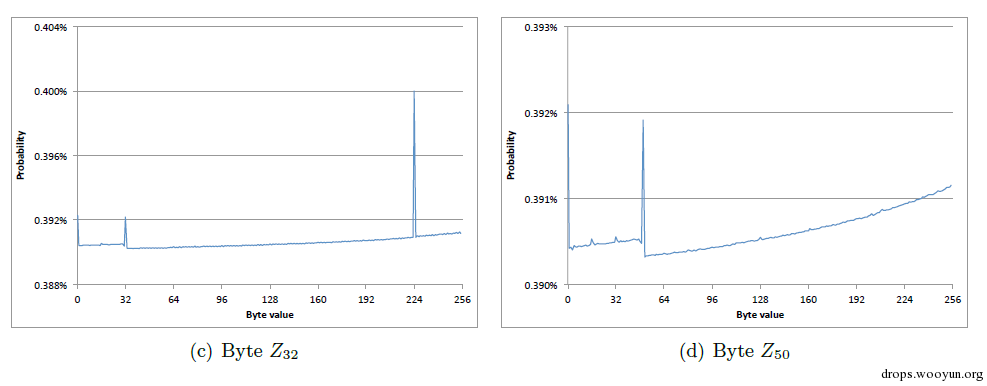## 2. 多字节偏移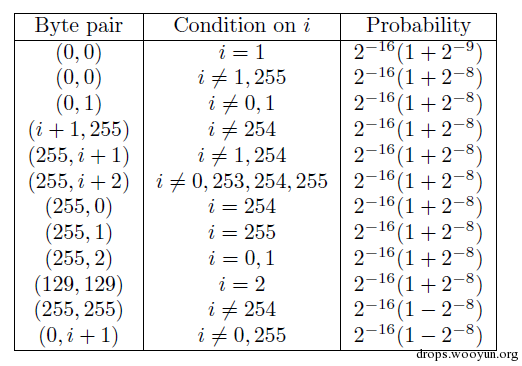## 3. RC4NOMORE

2015年Mathy Vanhoef及Frank Piessens发表了对RC4新的攻击方法，他们发现了新的偏移，只需要约9*2^27个密文就可以使cookie破解成功率达到94%，破解时间为75小时。这是第一个被证实可行的此类攻击。他们的论文《All Your Biases Belong to Us: Breaking RC4 in WPA-TKIP and TLS》发表在USENIX Security2015，并被评为Best Student Paper

# 0x03 概率

“随机抛n次硬币，求恰好出现m次正面向上的概率”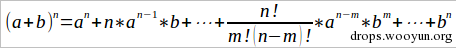“随机抛n次正方体骰子，点数1-6出现的次数分别为(x1,x2,x3,x4,x5,x6)的概率是多少,其中x1+x2+x3+x4+x5+x6=n”